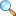# Chapter 01: Real numbers, limits and continuityNotes of the book Calculus with Analytic Geometry written by Dr. S. M. Yusuf and Prof. Muhammad Amin, published by Ilmi Kitab Khana, Lahore - PAKISTAN. The notes of this chapter is written by Prof. Muhammad Farooq, Punjab College, Sargodha.

• Articles of Exercise 1.1
• Rational numbers, Irrational numbers, Real numbers, Complex numbers
• Properties of real numbers, Order properties of $\mathbb{R}$
• Absolute value or modules of a $\mathbb{R}$
• The completeness property of $\mathbb{R}$
• Upper bound, lower bound
• Real line, Interval
• Working rule for the solution of inequality
• Binary relation (B.R), Domain of B.R, Range of B.R
• Function, Onto or surjective funiton, (1-1) Function, Bijective Function
• Real valued function, Image of a function, Bracket function
• Articles of Exercise 1.2
• Finite limit at a finite point
• Left hand limit
• Right hand limit
• Articles of Exercise 1.3
• Continuity

Select the exercise below to view it. You can also download the PDF file of the respective exercise from the pages given below.

• Articles of Exercise 1.1 |View Online | Download PDF
• Solution of Exercise 1.1 |View Online | Download PDF
• bsc/notes_of_calculus_with_analytic_geometry/ch01-_real_numbers_limits_and_continuity
• Last modified: 18 months ago
• (external edit)